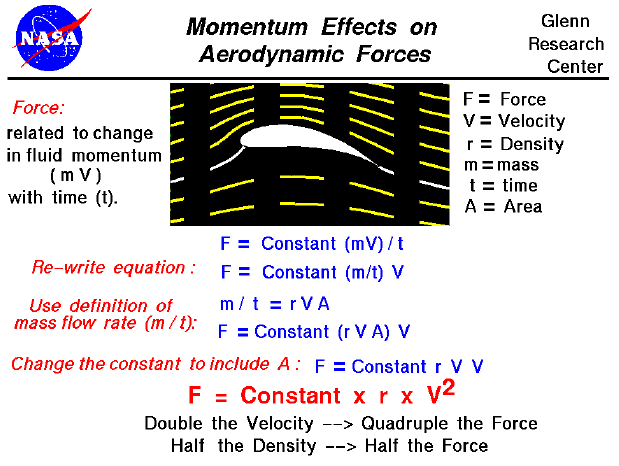Lift is created by deflecting a flow of air, and drag is generated on a body in a wide variety of ways. From Newton's Second Law of Motion, the aerodynamic forces on the body (lift and drag) are directly related to the change in momentum of the fluid with time. The fluid momentum is equal to the mass (m)times the velocity (V) of the fluid. Since the air moves, defining the mass is tricky. If the mass of fluid were brought to a halt, it would occupy some volume in space. And we could define its density (r) to be the mass divided by the volume. But since the fluid is moving, we must consider, instead, the mass flow rate, which is the amount of mass passing a given point during some time (mass/time). We can relate the mass flow rate to the density mathematically. The mass flow rate (mass/time) is equal to the density (mass/volume) times the velocity (length/time) times the area. With knowledge of the mass flow rate, we can express the aerodynamic force as equal to the mass flow rate times the velocity. A quick units check shows that the force (mass times the velocity divided by the time) is equal to the mass flow rate (mass divided by time) times the velocity. Therefore, the aerodynamic force is equal to a constant times the density times the velocity times the area times the velocity. Combining the velocity dependence and absorbing the area into the constant, we find that the aerodynamic force is equal to a constant times the density times the velocity squared.

Effect of Velocity on Aerodynamic Forces

The velocity used in the aerodynamic equation is the relative velocity between an object and the flow. The aerodynamic force depends on the square of the velocity. Doubling the velocity quadruples the force.

Effect of Air Density on Aerodynamic Forces

The aerodynamic force depends linearly on the density of the air. Halving the density halves the force. As altitude increases, the air density decreases. This explains why airplanes have a flight ceiling, an altitude above which it cannot fly. As an airplane ascends, a point is reached where there is not enough air mass to generate enough lift to overcome the airplane's weight. The relation between altitude and density is a fairly complex exponential.

FoilSim Applet

You can investigate the effect of momentum on lift by using the FoilSim II Java Applet. Set a small angle of attack using the slider, then vary the "Speed" and "Altitude." Try doubling the speed and notice the effect on lift. Change the altitude until the air density is half of its previous value. What happened to the lift? You can use the browser "Back" button to return to this page. If your browser does not support JAVA, or you just want your own copy of FoilSim to play with, you can download it at no charge.

Guided Tours

Back to top

Go to...

Beginner's Guide Home Page

byTom Benson
Please send suggestions/corrections to: benson@grc.nasa.gov